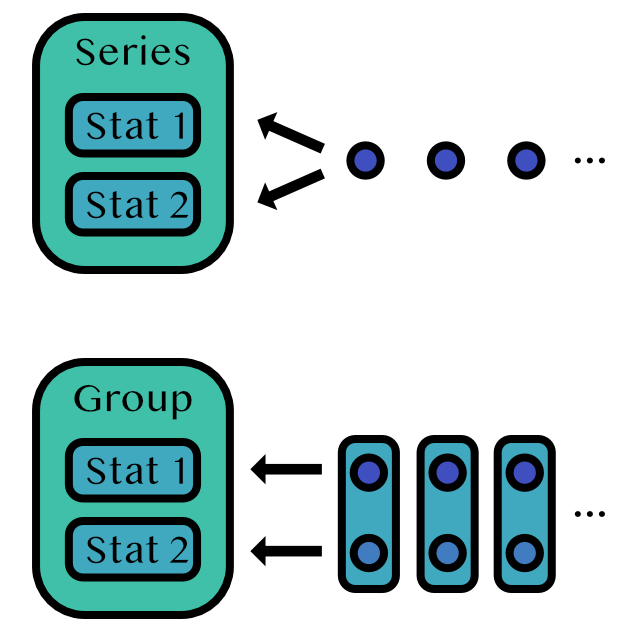# Collections of Stats

There are a few special OnlineStats that group together other OnlineStats: Series and Group.## Series

A Series tracks stats that should be applied to the same data stream.

y = rand(1000)

s = Series(Mean(), Variance())

fit!(s, y)
Series
├─ Mean: n=1000 | value=0.497399
└─ Variance: n=1000 | value=0.0891061


### FTSeries

An FTSeries tracks stats that should be applied to the same data stream, but filters and transforms (hence FT) the input data before it is sent to its stats. This is useful for things like removing missing values.

T = Union{Missing,Number}

s = FTSeries(T, Mean(), Variance(); filter = !ismissing, transform = abs)

fit!(s, [-1, missing])
FTSeries
├─ Mean: n=1 | value=1.0
└─ Variance: n=1 | value=1.0


## Group

A Group tracks stats that should be applied to different data streams.

g = Group(Mean(), CountMap(Bool))

itr = zip(randn(100), rand(Bool, 100))

fit!(g, itr)
Group
├─ Mean: n=100 | value=-0.12751
└─ CountMap: n=100 | value=OrderedCollections.OrderedDict{Bool, Int64}(1=>39, 0=>61)